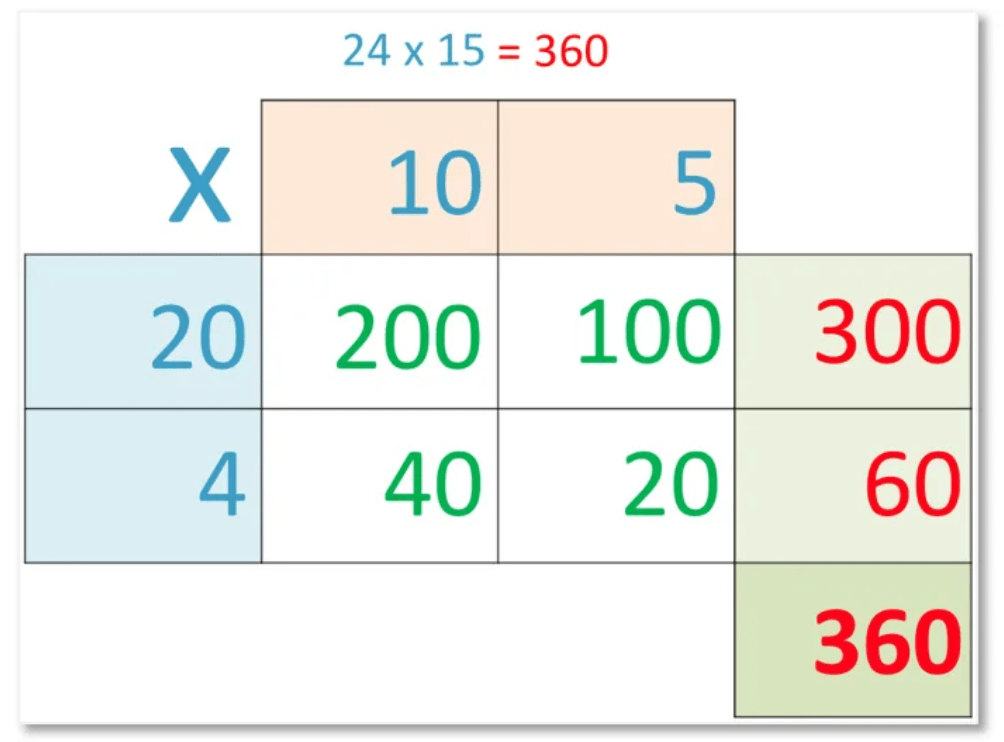# How to do Long Multiplication

How to do Long Multiplication• Write the larger number above the smaller number with a multiplication sign next to the smaller number.
• Multiply the ones digit of the top number by the ones digit of the bottom number and write this answer below.
• Multiply the tens digit of the top number by the ones digit of the bottom number and write this to the left of the first answer.
• If any of the answers have two digits, write the first digit in the next box to the left, smaller than the other digits.
• Now write a 0 digit below these first answers.
• Multiply the ones digit of the top number by the tens digit of the bottom number and write this answer next to the 0.
• We write it next to a zero because we are multiplying by tens digit and we need to make the result 10 times larger.
• Multiply the tens digit of the top number by the tens digit of the bottom number and write this result to the left of the previous answer.
• Again, if any of these answers contain 2 digits, write the first digit in the box to the left, smaller than the other digits.
• Now we add the two lines of working out to get our final answer.
Multiply the numbers digit by digit, writing a zero first if the bottom digit is in the tens column.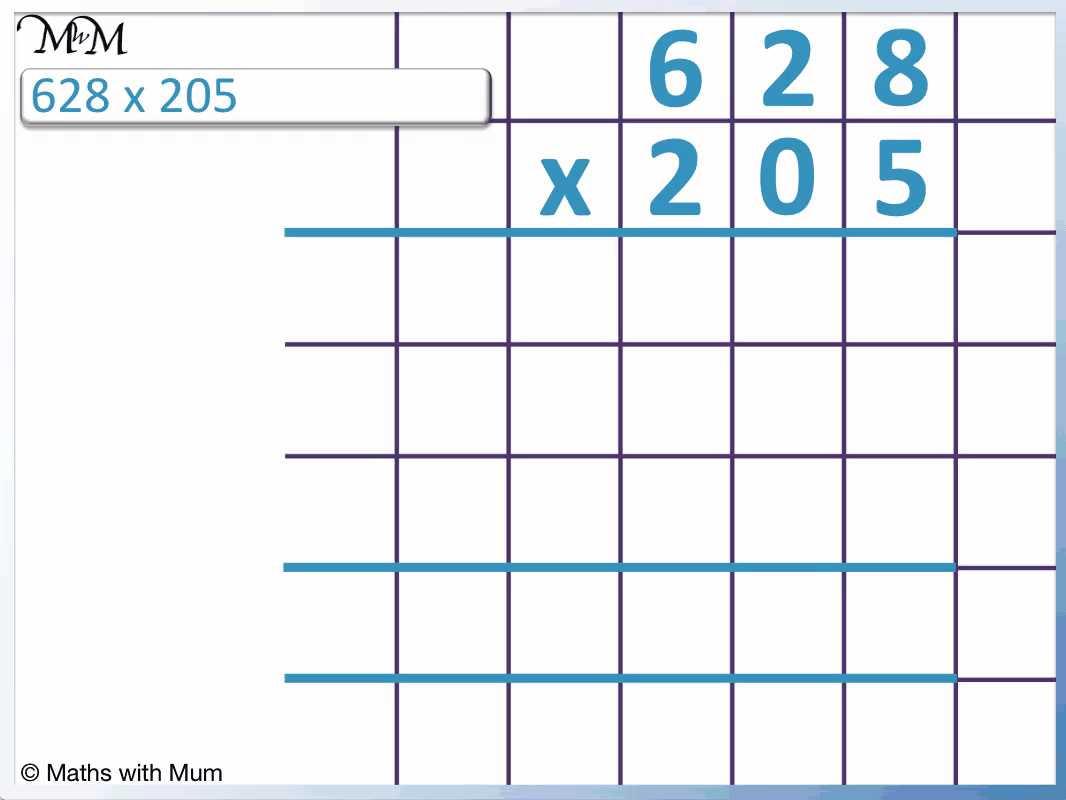• We multiply the digits of the top number by the digits of the bottom number.
• We put a 0 on the end if the bottom digit is in the tens column.
• We put two 0’s on the end if the bottom digit is in the hundreds column.
• 8 × 5 = 40, so we write a 0 below and a small 4 in the box to its left.
• 2 × 5 = 10 but we must add the 4 that we carried to make 14. We write a 4 and carry the 1.
• 6 × 5 = 30 but we must add the 1 that we carried to make 31. We write a 1 and carry the 3
• So far we have found that 628 × 5 = 3140.
• Now we multiply 628 by the 0 in the tens column.
• 628 × 0 is just 0 so we do not need to write anything and we move on to look at 628 × 200.
• The digit of 2 represents 200 and so, we write two 0’s in so that we can multiply 628 × 2 instead.
• 8 × 2 = 16, so we write the 6 next to the two 0’s and then carry the 1.
• 2 × 2 = 4 but we must add the 1 that we carried to make 5. We write the 5.
• 6 × 2 = 12, so we write the 2 and carry the 1.
• We can see that 628 × 200 = 125600.
• We now add the results of 628 × 5 and 628 × 200 to find the answer to 628 × 205.
• 3140 + 125600 = 128740 and so, 628 × 205 = 128740.#### Long Multiplication with 3-Digit Numbers# Long Multiplication

## What is Long Multiplication?

Long multiplication is a method used for multiplying two numbers that both contain more than 1 digit. The method involves multiplying each digit in each number separately and then adding the result at the end.

We teach the long multiplication method when the numbers being multiplied have more than 1 digit.

## Long Multiplication of 2-Digit Numbers

To do long multiplication of 2-digit numbers, follow these steps:

1. Multiply the ones digit of the top number by the ones digit of the bottom number.
2. Multiply the tens digit of the top number by the ones digit of the bottom number and write this alongside the answer to step 1 to make one number.
3. Write a 0 below.
4. Multiply the ones digit of the top number by the tens digit of the bottom number and write this answer to the left of the 0.
5. Multiply the tens digit of the top number by the tens digit of the bottom number and write this alongside the answer to step 4 to make one number.
6. Add the two lines of working out to get the final answer.

For example, here is the long multiplication of the two 2-digit numbers 84 × 57.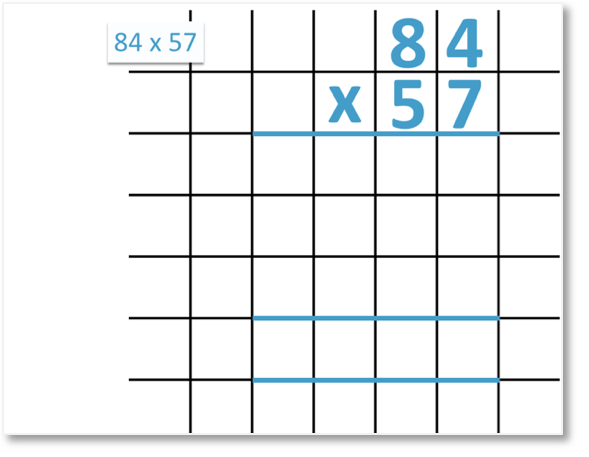To set out long multiplication, write the larger number number above the smaller number with each digit in a separate grid of the paper.

Step 1 is to multiply the ones digit of the top number by the ones digit of the lower number.

We multiply 4 by 7 to get 28. In long multiplication, only write one digit per grid on the paper.

We write the 8 in the box below the ones column and carry the 2 tens by writing them in the box to the left, smaller than the other numbers.Step 2 is to multiply the tens digit of the top number by the ones digit of the bottom number.

8 × 7 = 56.

We must add the 2 that we carried from the previous calculation.

56 + 2 = 58, so we write 58 tens to the left of the 8 written previously.We have multiplied the top number by the ones digit of the bottom number.

84 × 7 = 588.

Step 3 is to write a zero in the line below. We do this because we are about to multiply the top number by the tens digit of the bottom number.

Because we are multiplying by the tens digit, we write the 0 first to provide the effect of multiplying by 10.

Here we are multiplying by 50, not 5. By writing a 0, we can multiply by 5 instead, which makes our calculations easier.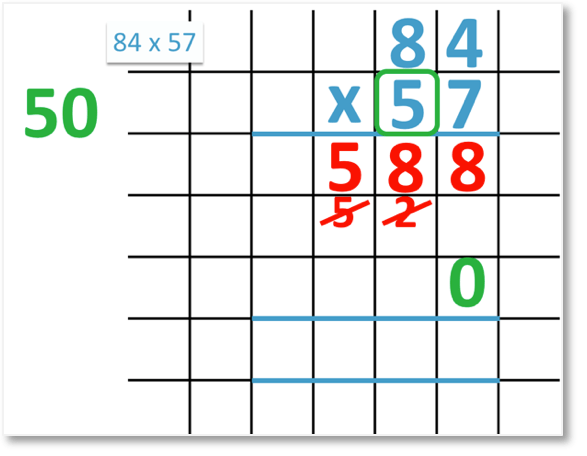Step 4 is to multiply the ones digit of the top number by the tens digit of the bottom number.

4 × 5 = 20. We write the 0 and carry the 2.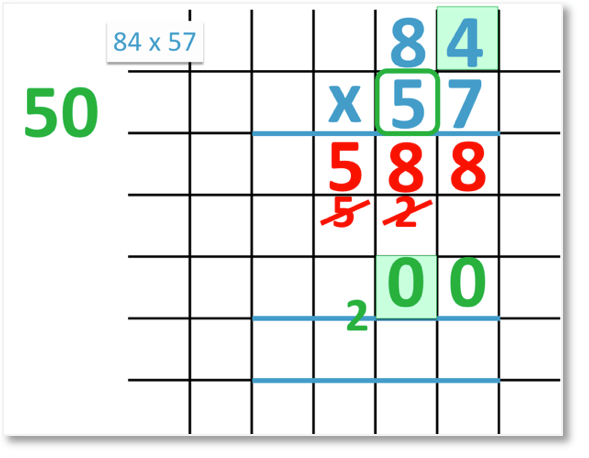Step 5 is to multiply the tens digit of the top number by the tens digit of the bottom number.

8 × 5 = 40. We Add this to the 2 that we carried to make 42.84 × 50 = 4200.

Step 6 is to add the two lines of working.

We add 84 × 7 and 84 × 50 to find the answer to 84 × 57.

588 + 4200 = 4788 and so, 84 × 57 = 4788.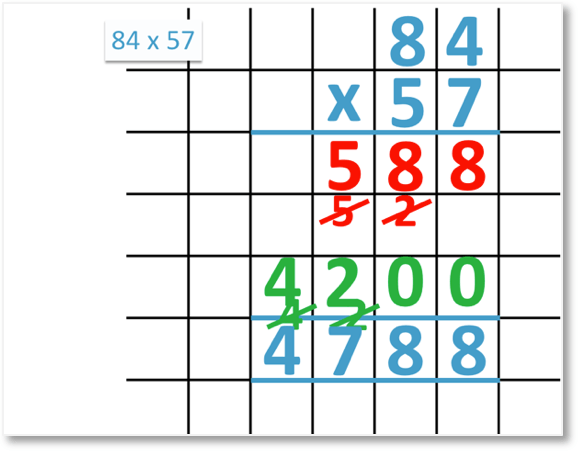## Long Multiplication of 3-Digit Numbers

To do long multiplication of 3-digit numbers, multiply all 3 digits of both numbers together individually. When multiplying by the tens digit of the bottom number, write a 0 on the end of your answer. When multiplying by the hundreds digit of the bottom number, write two 0’s on the end of your answer. Add the three numbers together to obtain the final answer.

For example, here is the long multiplication of the two three digit numbers 265 × 374.

To set out long multiplication, write one number above the other with a separate digit in each box.We will first multiply 265 by the ones digit of the bottom number.Multiplying 5 × 4 = 20, we write the 0 and carry the 2.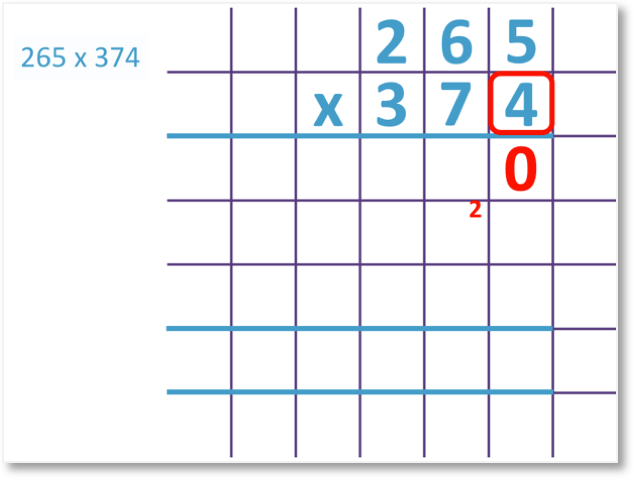Next we multiply the 6 in the tens column by the 4.

6 × 4 = 24. We add this to the 2 carried previously to make 26. We write the 6 and carry the 2.Next we multiply the 2 in the hundreds column of the top number by the 4.

2 × 4 = 8. We add this to the 2 carried previously to make 10. We write the 0 an carry the 1 to the next column to the left.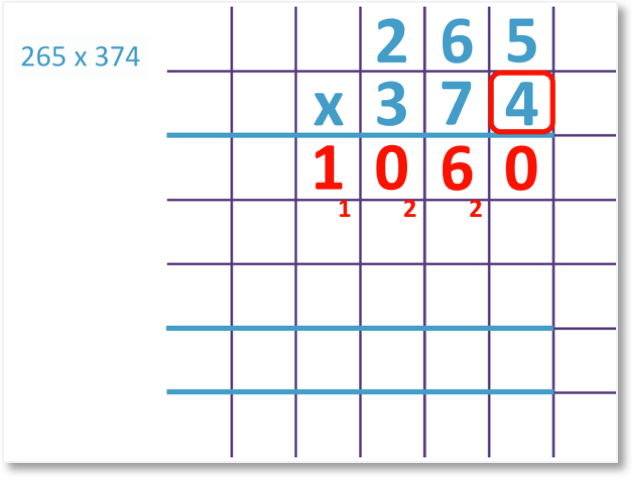265 × 4 = 1060.

Next we multiply the digits in the top number by the tens column digit of the bottom number.

Because we are multiplying by the tens digit, we first write a zero to provide the effect of multiplying by 10.

We are now multiplying 265 by 70. By writing the 0 in place, we can simply multiply 265 by 7 instead.We multiply 5 by 7 to get 35. We write the 5 and carry the 3.Next we multiply the 6 in the tens column of the top number by 7.

6 × 7 = 42 and when we add the 3 carried previously, we get 45. We write the 5 and carry the 4.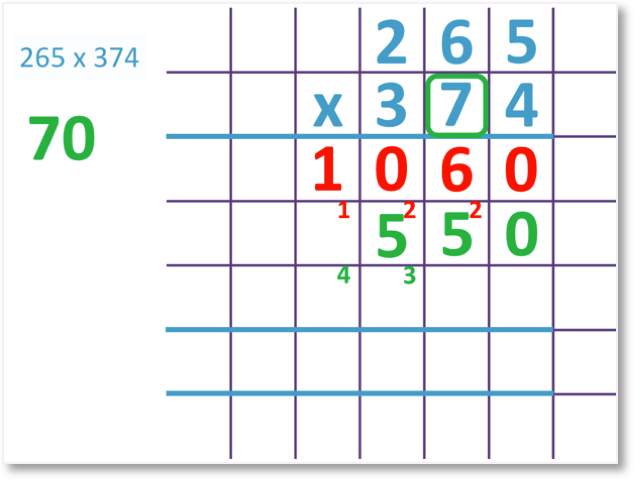Next we multiply the 2 in the hundreds column by the 7.

2 × 7 = 14. We add this to the 4 that we carried to get 18. We write the 8 and carry the 1.265 × 70 = 18550.

Next we multiply 265 × 300.

Because the digit of 3 represents 300, we write two 0 digits in the next line of working out.

Adding two 0 digits to the end of a number has the effect of multiplying by 100. This way, we can multiply by 3 instead of 300, which keeps the calculation easier to manage.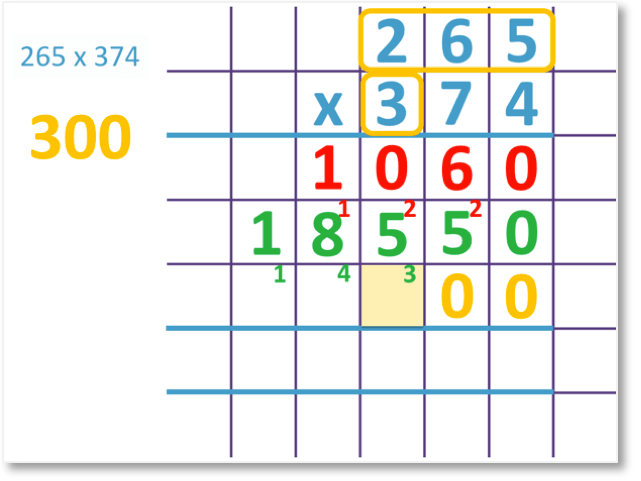We multiply the 5 in the ones column of the top number by 3 to get 15. We write the 5 and carry the 1.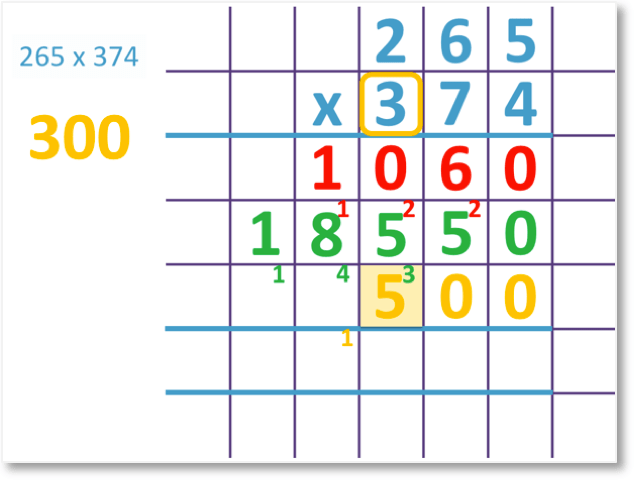Next we multiply the 6 in the tens column of the top number by 3 to get 18. We add this to the 1 that we carried to get 19. We write the 9 and carry the 1.Next we multiply the 2 in the hundreds column of the top number by 3 to get 6. We add this to the 1 that we carried to make 7.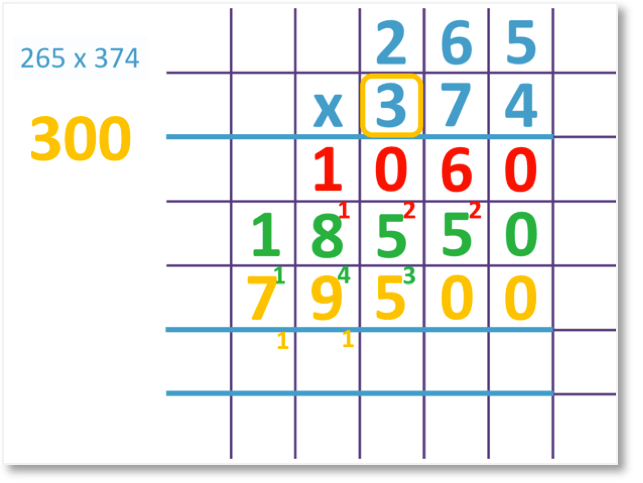We can see that 265 x 300 = 79500.

The final step is to add the three lines of working out.

Adding 265 x 4, 265 x 70 and 265 x 300 we will find the answer to 265 x 374.

We add the three numbers column by column from right to left.

In the ones column we have 0.

Adding the 6 and 5 in the tens column, we have 11. We write 1 and carry 1.

Adding the 0 + 5 + 5 in the hundreds column to the 1 that we carried, we get 11. We write 1 and carry 1.

Adding the 1 + 8 + 9 in the thousands column to the 1 that we carried, we get 19. We write the 9 and carry the 1

Adding the 1 + 7 in the ten-thousands column to the 1 that we carried, we get 9, which we write down.1060 + 18550 + 79500 = 99110 and so, 265 x 374 = 99110.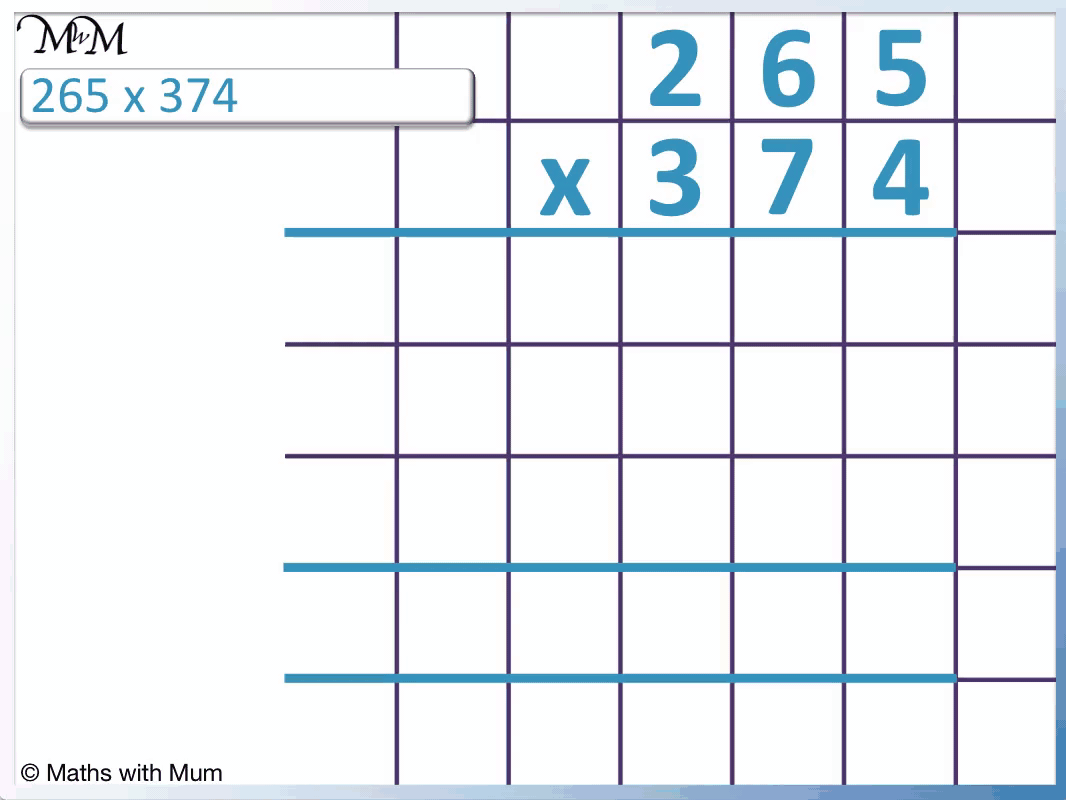## How to do Long Multiplication

To do long multiplication, follow these steps:

1. Write the larger number above the smaller number.
2. Multiply each digit of the top number by the ones digit of the bottom number to create a number to write in the first line of working out.
3. Write a zero in the second line of working out below, in the ones column.
4. Multiply each digit of the top number by the tens digit of the bottom number to create a number to write to the left of the 0 in the second line of working out.
5. Write two 0’s in the third line of working out below, in the ones column.
6. Multiply each digit of the top number by the hundreds digit of the bottom number to create a number to write to the left of the two 0’s in the second line of working out.
7. Continue these steps if there are more digits in the bottom number, writing an extra 0 for each new line of working out.
8. Add the lines of working out to find the final answer below.

Here is an example of 287 x 35.We multiply 287 by 5 and then by 30 before adding the results together.

Multiplying the ones digits of both numbers, 5 x 7 = 35. We write the 5 and carry the 3.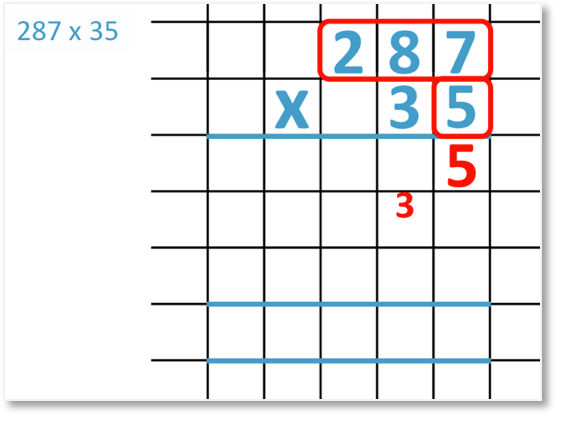Next we multiply the tens digit of the top number by the ones digit of the bottom number.

8 x 5 = 40. We add this to the 3 we carried to get 43. We write the 3 and carry the 4.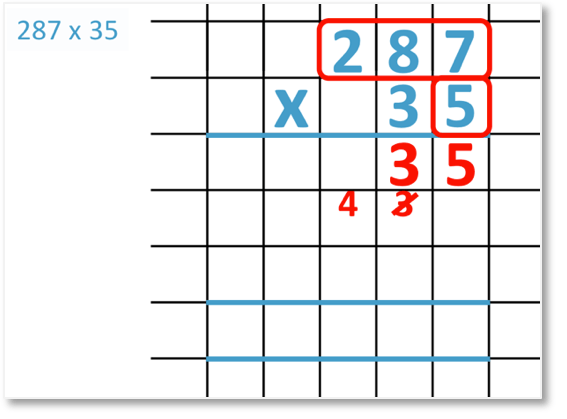Next we multiply the hundreds digit of the top number by the ones digit of the bottom number.

2 x 5 = 10. We add this to the 4 we carried to get 14. We write the 4 and carry the 1.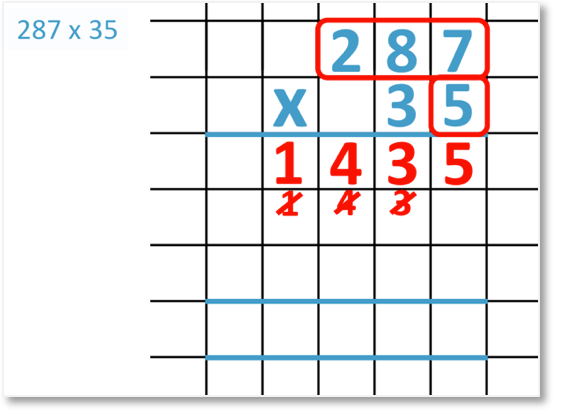287 x 5 = 1435.

We now multiply 287 by 30.

We write a 0 in the next line of working out to give the effect of multiplying by 10. This means that we only need to multiply 287 by 3 rather than 30.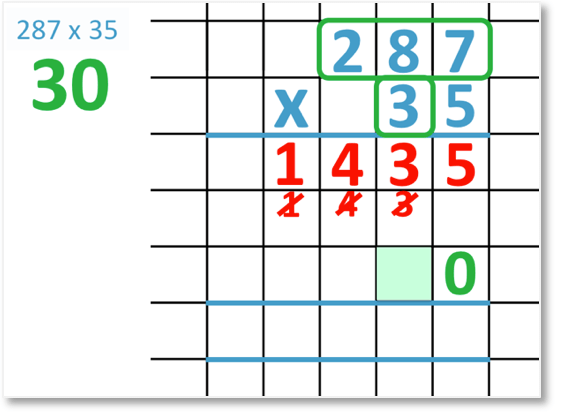7 x 3 = 21. We write the 1 and carry the 2.Next we multiply the tens digit of 287 by the 3.

8 x 3 = 24. We add this to the 2 that we carried to get 26. We write the 6 and carry the 2.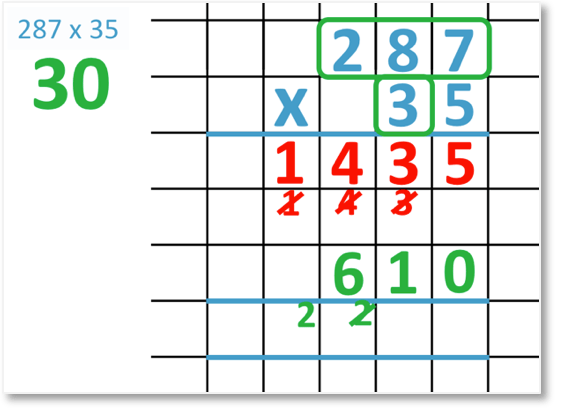Next we multiply the hundreds digit of 287 by the 3.

2 x 3 = 6 and so, we add this to the 2 we carried to get 8.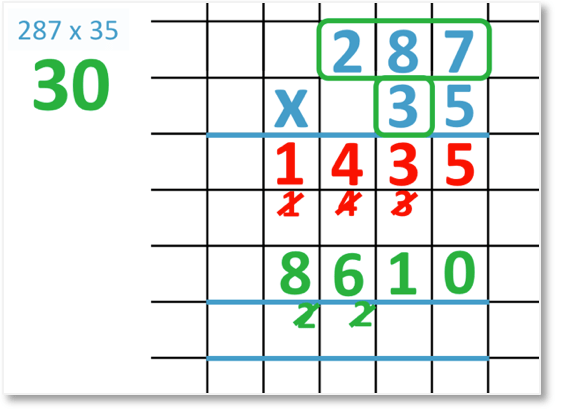287 x 30 = 8610.

We have calculated 287 x 5 and 287 x 30. We add these two lines of working to find 287 x 35.

In the ones column, 5 + 0 = 5.

In the tens column, 2 + 1 = 3.

In the hundreds column, 4 + 6 = 10. We write the 0 and carry the 1.

In the thousands column, 1 + 8 + the 1 we carried = 10. We write the 0 and carry the 1.

1425 + 8610 = 10045 and so, 287 x 35 = 10045.Now try our lesson on Grid Method Multiplication where we learn how to use the grid method to multiply numbers.